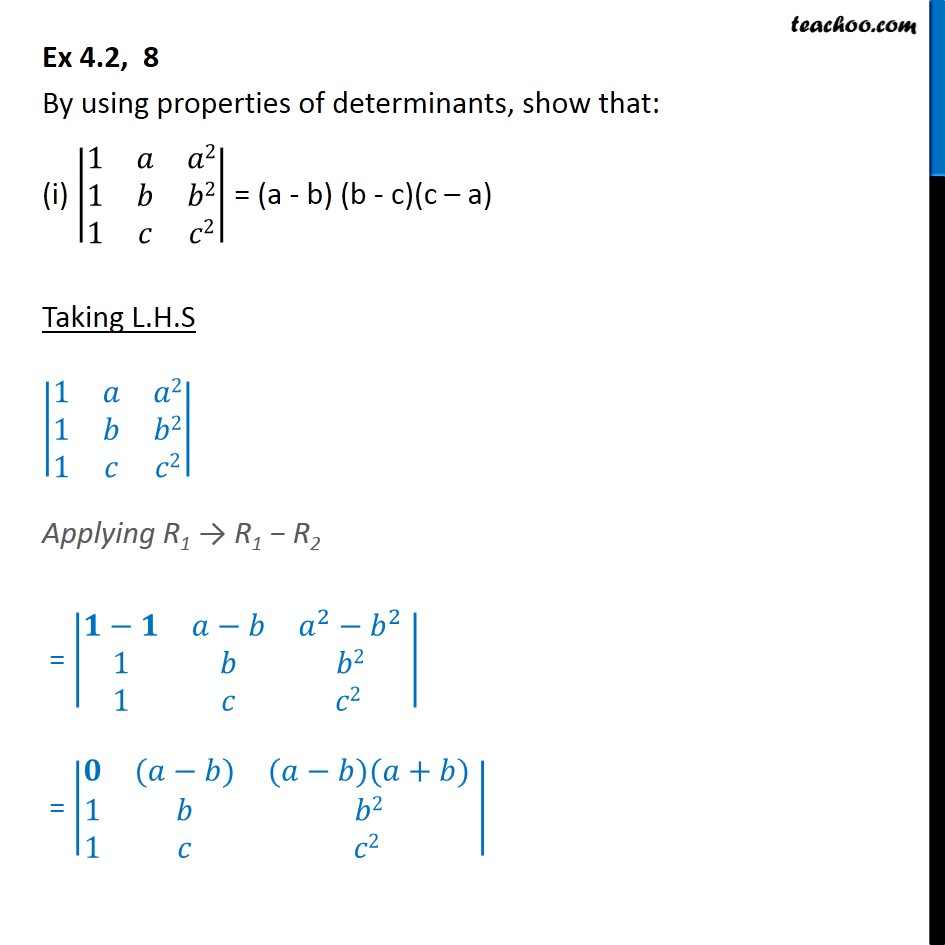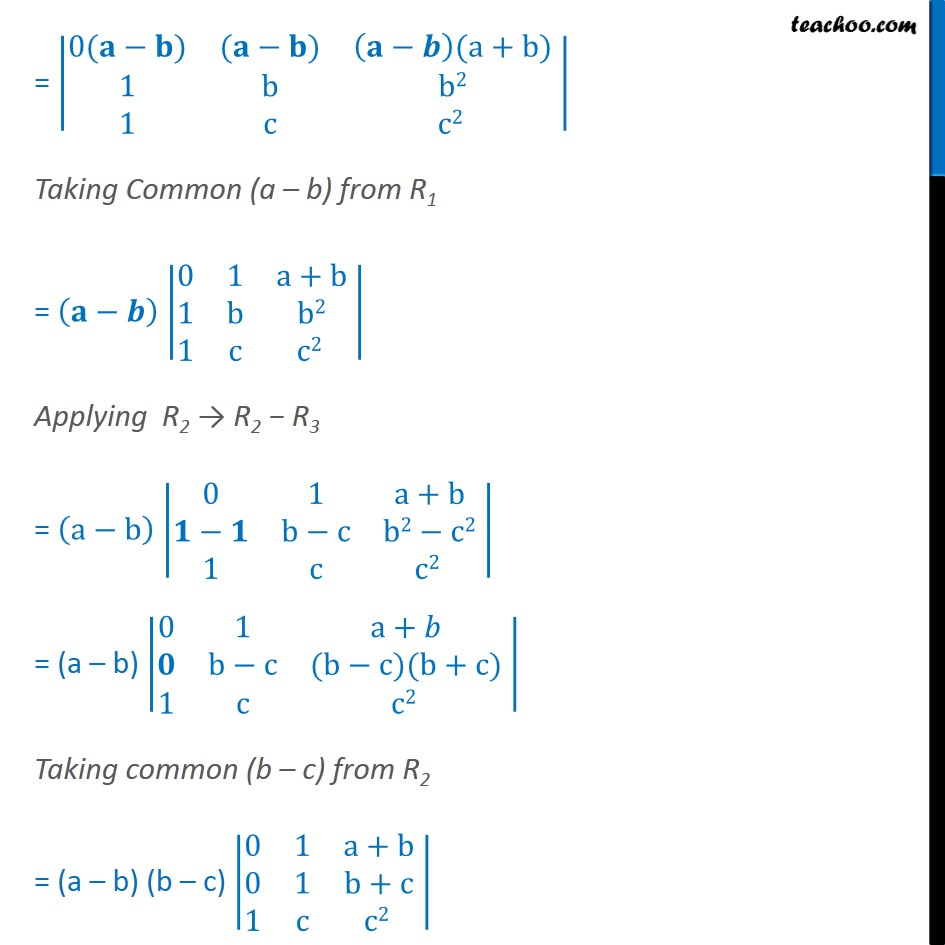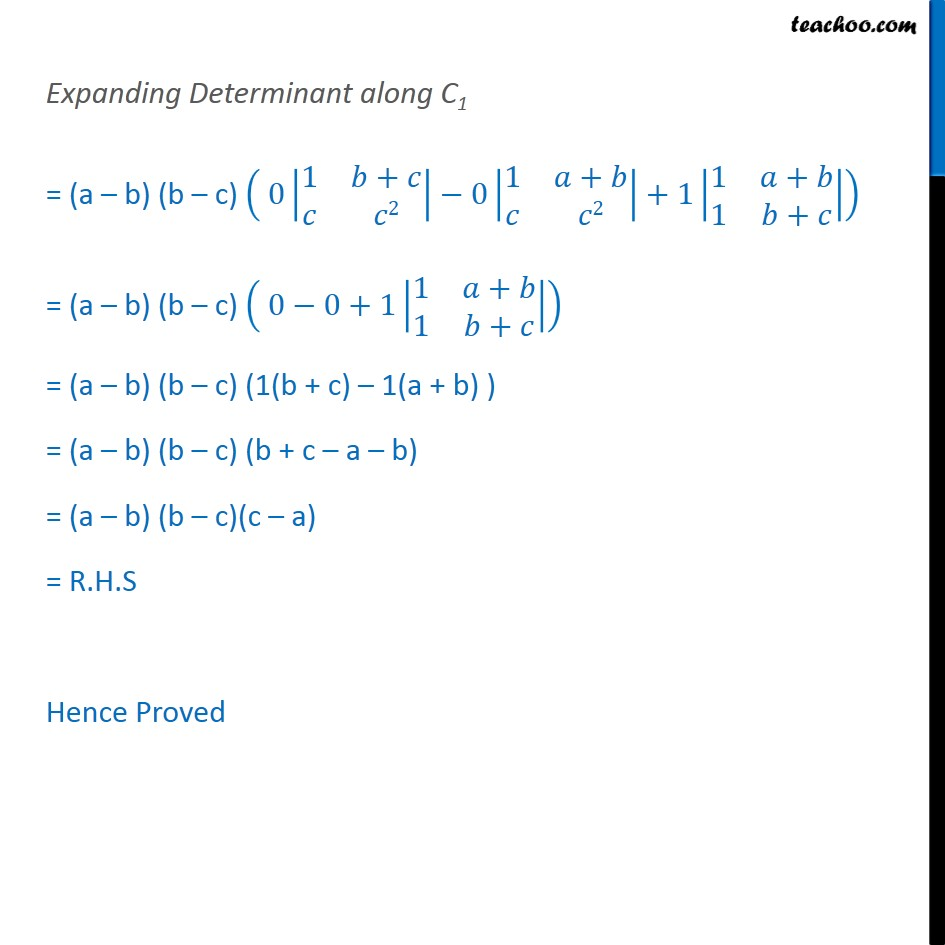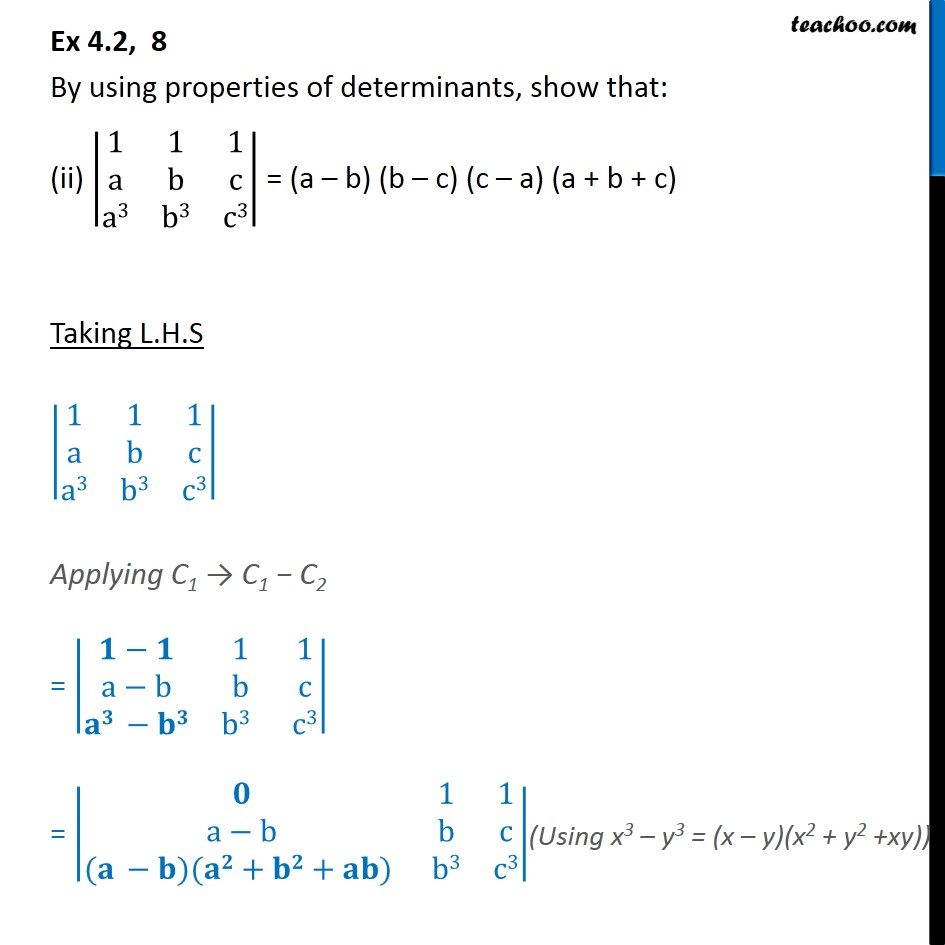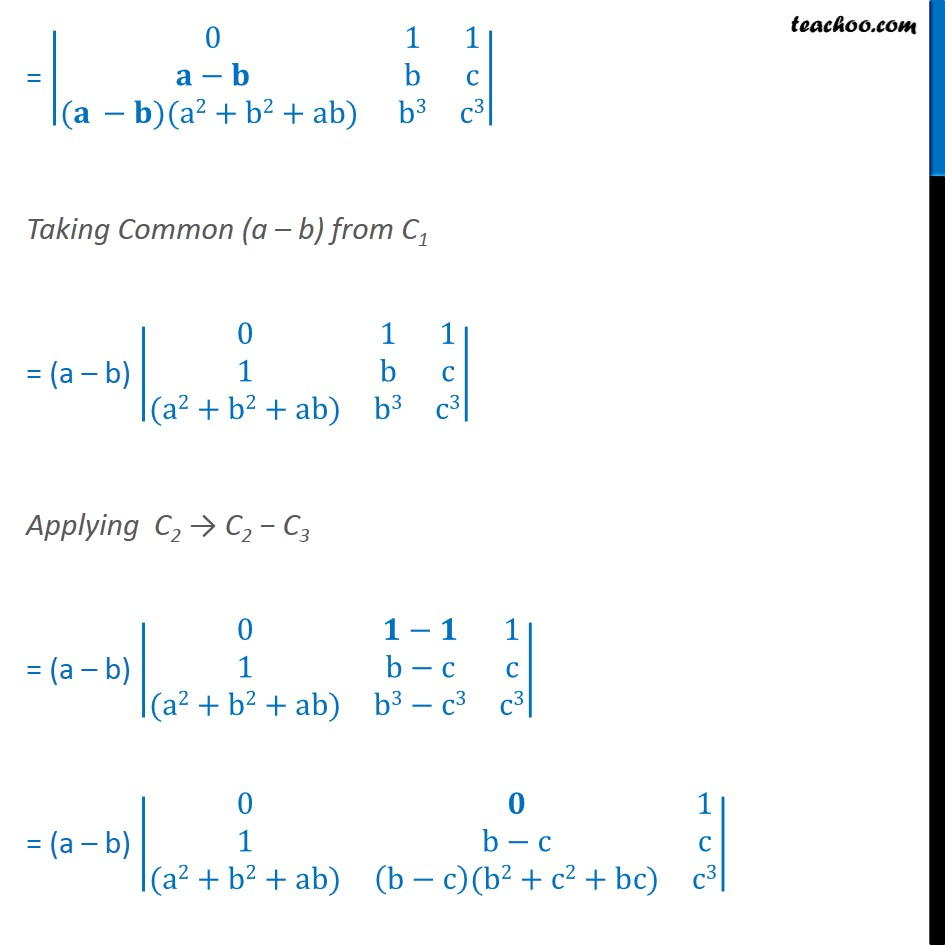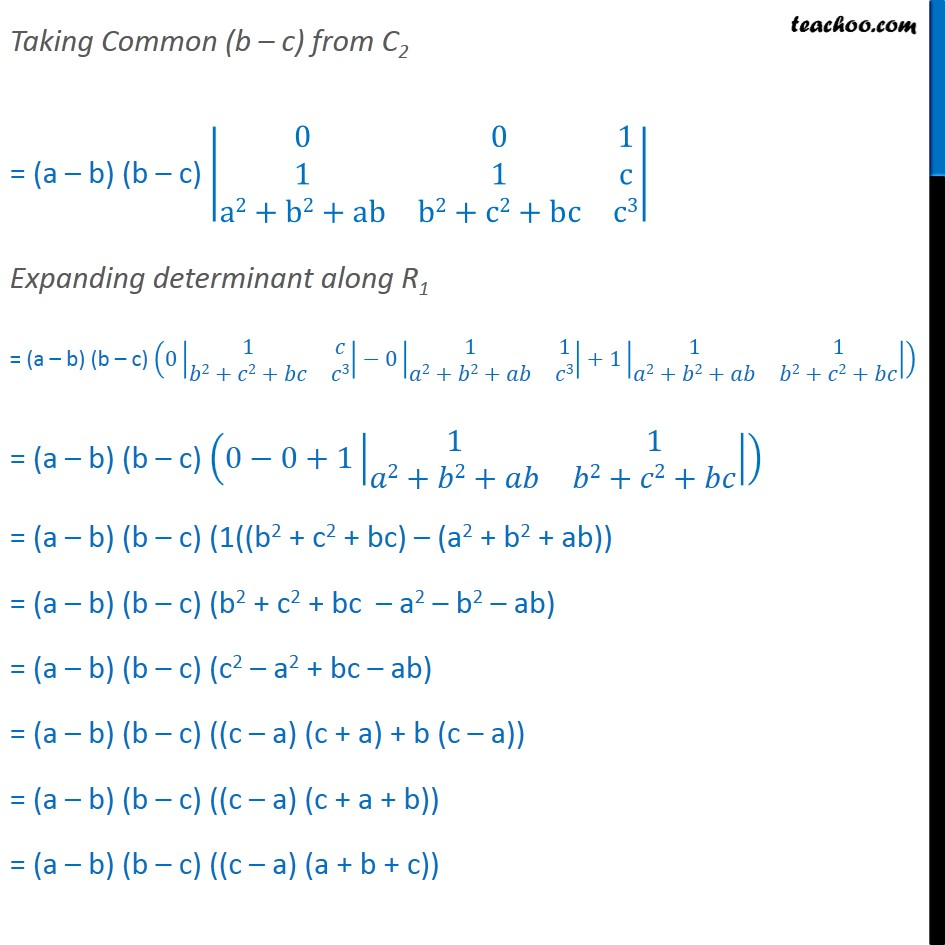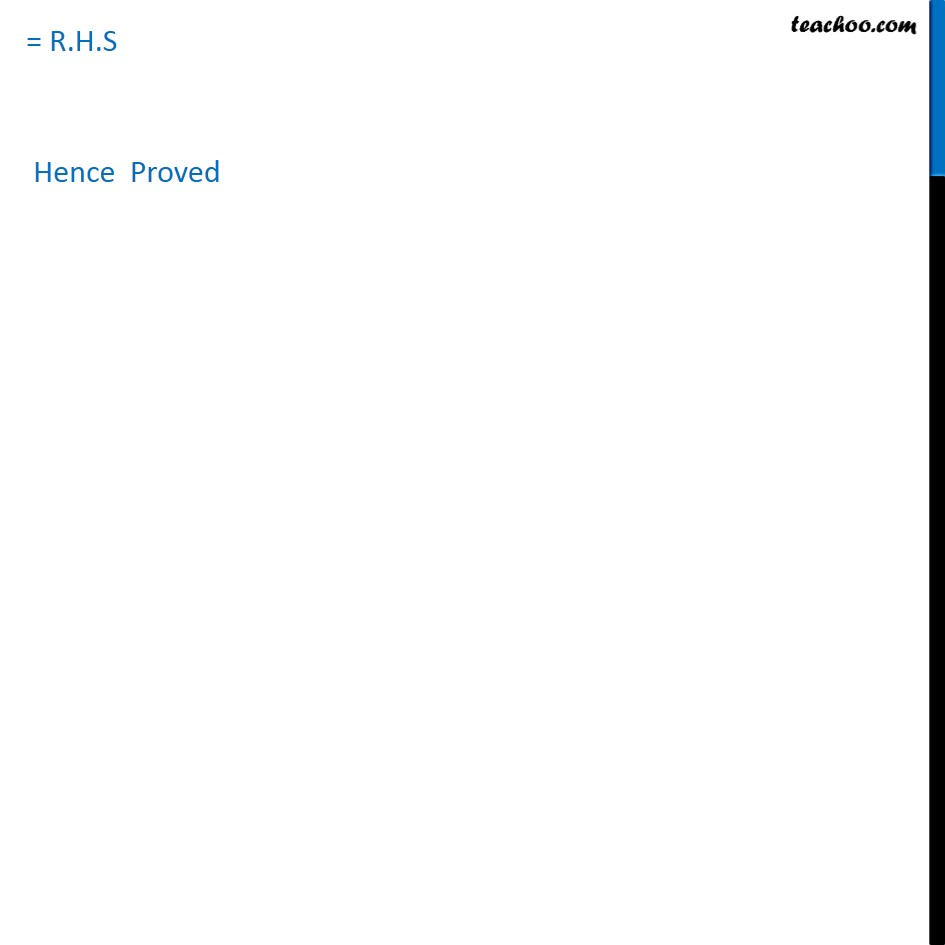1. Chapter 4 Class 12 Determinants
2. Concept wise
3. Whole row/column one

Transcript

Ex 4.2, 8 By using properties of determinants, show that: (i) 1 2 1 2 1 2 = (a - b) (b - c)(c a) Taking L.H.S 1 2 1 2 1 2 Applying R1 R1 R2 = 2 2 1 2 1 2 = ( ) ( )( + ) 1 2 1 2 = 0( ) ( ) (a+b) 1 b b2 1 c c2 Taking Common (a b) from R1 = 0 1 a+b 1 b b2 1 c c2 Applying R2 R2 R3 = a b 0 1 a+b b c b2 c2 1 c c2 = (a b) 0 1 a+ b c (b c)(b+c) 1 c c2 Taking common (b c) from R2 = (a b) (b c) 0 1 a+b 0 1 b+c 1 c c2 Expanding Determinant along C1 = (a b) (b c) 0 1 + 2 0 1 + 2 +1 1 + 1 + = (a b) (b c) 0 0+1 1 + 1 + = (a b) (b c) (1(b + c) 1(a + b) ) = (a b) (b c) (b + c a b) = (a b) (b c)(c a) = R.H.S Hence Proved Ex 4.2, 8 By using properties of determinants, show that: (ii) 1 1 1 a b c a3 b3 c3 = (a b) (b c) (c a) (a + b + c) Taking L.H.S 1 1 1 a b c a3 b3 c3 Applying C1 C1 C2 = 1 1 a b b c b3 c3 = 1 1 a b b c ( )( + + ) b3 c3 = 0 1 1 b c ( )(a2+b2+ab) b3 c3 Taking Common (a b) from C1 = (a b) 0 1 1 1 b c (a2+b2+ab) b3 c3 Applying C2 C2 C3 = (a b) 0 1 1 b c c (a2+b2+ab) b3 c3 c3 = (a b) 0 1 1 b c c (a2+b2+ab) b c (b2+c2+bc) c3 Taking Common (b c) from C2 = (a b) (b c) 0 0 1 1 1 c a2+b2+ab b2+c2+bc c3 Expanding determinant along R1 = (a b) (b c) 0 1 2+ 2+ 3 0 1 1 2+ 2+ 3 +1 1 1 2+ 2+ 2+ 2+ = (a b) (b c) 0 0+1 1 1 2+ 2+ 2+ 2+ = (a b) (b c) (1((b2 + c2 + bc) (a2 + b2 + ab)) = (a b) (b c) (b2 + c2 + bc a2 b2 ab) = (a b) (b c) (c2 a2 + bc ab) = (a b) (b c) ((c a) (c + a) + b (c a)) = (a b) (b c) ((c a) (c + a + b)) = (a b) (b c) ((c a) (a + b + c)) = R.H.S Hence Proved

Whole row/column one